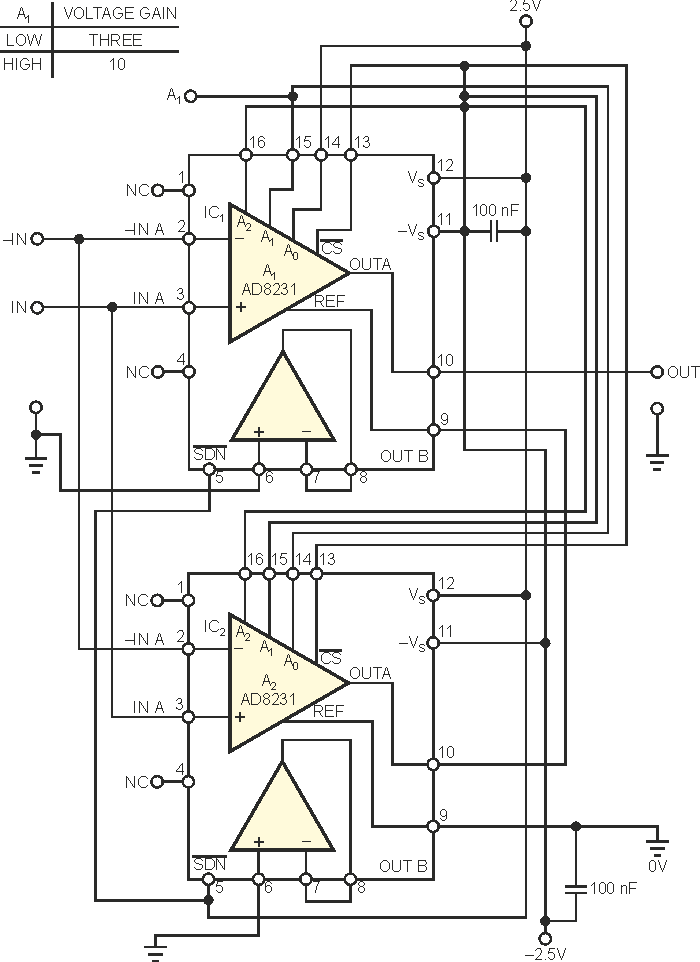# Digitally Programmable Instrumentation Amplifier Offers Autozeroing

The current trend in advanced instrumentation amps is to use no external resistors. In these amplifiers, a gain-control word, comprising a binary-coded one, sets the voltage gain. Several integer gains within one to 1000 are currently available; however, this range does not yet include a gain of three. Although external-resistor-free amplifiers with a gain of three are available, they are neither instrumentation amps nor autozeroed devices (Reference 1). These features are essential in applications requiring accurate processing of low-level voltages. You can use the circuit in Figure 1 for applications requiring instrumentation amps having voltage gains of three or 10 and the ability to process voltages as low as 1 mV.Figure 1. The autozeroed instrumentation amp, digitally programmable for voltage gains of three and 10, can help you to overcome the current inavailability of monolithic ICs for this task.

This design achieves a voltage gain of three by using the “algorithm” of 3 = 2 + 1. The circuit comprises two units of the Analog Devices digitally gain-programmable, autozeroed AD8231 instrumentation amp. These ICs have voltage gains that are programmable as powers of two – that is, one, two, four … 128 (Reference 2). Amplifier A1 in IC1 is preset to provide a gain of two, and auxiliary amp A2 in IC2 is preset to a gain of one. The noninverting and inverting inputs of A1 and A2 connect together. The output of A2 connects with reference input REF1, and reference input REF2 serves as a freely usable reference. You can thus calculate the output voltage as

VOUT = VOUT1 + VREF1 = VOUT1 + VOUT2 = 2ΔVIN + ΔVIN = 3ΔVIN,

where ΔVIN is the input-difference voltage.

Similarly, you can achieve a voltage gain of 10 according to a symbolic formula of 10 = 8 + 2. This time, A1 has a voltage gain of eight, and A2 has a gain of two. Using Reference 2, you can derive that, for gains of both three and 10, A1A1 = A0A2. Therefore, the gain-control pins connect and remain low for a gain of three, and the high at these pins sets the gain to 10. Note that three approaches the square root of 10, or approximately 3.16. You can therefore consider it as roughly the geometric center of a decade.

## References

1. Štofka, Marian, “Gain-of-three amplifier requires no external resistors”, EDN, Aug 16, 2006, pg 74.
2. “Zero Drift, Digitally Programmable Instrumentation Amplifier, AD8231,” Analog Devices Inc, 2007.

EDN